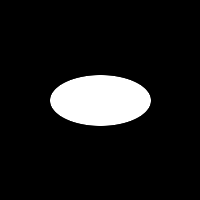## MOMENTS

 Compute shift, scale and rotation invariant image moments as well as elliptical shape descriptors

 Image And List of Moments Original Image Image Creation Parameters Moment List and Ellipse Shape DescriptorsX Center of Mass (XCOM) = 69.13896179 Y Center of Mass (YCOM) = 57.86068726 Ellipse Angle (radians) = 0.3220438063 Ellipse Angle (degrees) = 18.4517498 Ellipse Semi-Major Axis = 76.38931274 Ellipse Semi-Minor Axis = 71.42292786 Ellipse Eccentricity = 0.2549786866 Ellipse Intensity = 134.8245239White Ellipse: X,Y Center: 100,100 Semi-Major Axis: 50 Semi-Minor Axis: 25 Rotation: 0 degrees X Center of Mass (XCOM) = 100.0000687 Y Center of Mass (YCOM) = 100.0000229 Ellipse Angle (radians) = -1.268604365e-06 Ellipse Angle (degrees) = -7.268567424e-05 Ellipse Semi-Major Axis = 50.3578949 Ellipse Semi-Minor Axis = 25.35848236 Ellipse Eccentricity = 0.7045813203 Ellipse Intensity = 254.9430695White Rectangle: X,Y Center: 138,138 Width Axis: 50 Height: 25 Rotation: 30 degrees X Center of Mass (XCOM) = 137.5 Y Center of Mass (YCOM) = 137.5 Ellipse Angle (radians) = 0.5235797763 Ellipse Angle (degrees) = 29.9989109 Ellipse Semi-Major Axis = 57.73477936 Ellipse Semi-Minor Axis = 28.87569618 Ellipse Eccentricity = 0.707005024 Ellipse Intensity = 243.4593353